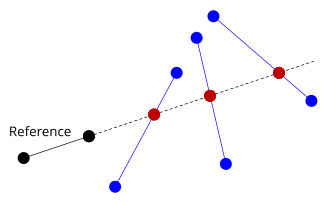# Finding the crossing points of two lines

This is a task that has come up for me many times when creating algorithms for images analysis. Searching the web will invariably turn up the y = Mx + C solution. This is sub-optimal in two ways. First, it has big problems with vertical lines. As the line tends to vertical then m will tend to infinity. This Is Not Good. Secondly, getting any sort of accuracy is impossible without using floating point or large scaling factors.

The code I was modifying at the time was using the y = Mx + C method. It was fairly complex and had to check for lines that were nearly vertical, and flip the axes. It had bugs. I had this gut feeling that must be a better way to solve this, without all of the messing about with edge cases.

Finally after a bit of Googling I came across the lesser used form for describing lines, C = Ax + By. Having lines described in this slightly different form allows a method to find crossing points that is much more code friendly.

Most image processing algorithms I have created work perfectly fine when pixel coordinates are restricted to integral values, allowing integer maths for the following example.

Take two lines, L1 = x1,y1 to x2,y2 and L2 = x3,y3 to x4,y4.Calculate the values of A, B and C for each line.

For L1:
A1 = y2 – y1
B1 = x1 – x2
C1 = (A1 * x1) + (B1 * y1)

And similarly for L2:

A2 = y4 – y3
B2 = x3 – x4
C2 = (A2 * x3) + (B2 * y3)

Next, work out the determinant.

D = (A1 * B2) – (A2 * B1)

If D is zero then the lines are parallel and there is no crossing point to be found.

If D is not zero then the coordinates of the crossing point may be found thus:

X = ((B2 * C1) – (B1 * C2)) / D
Y = ((A1 * C2) – (A2 * C1)) / D

The two lines do not have to actually cross for this to work. This makes it a great way to find all of the crossing points relative to a reference line.Next time I will show you how this technique can allow you to scan a rectangular region that is at an arbitrary angle.